Direct leveling by traversing

# Direct leveling by traversingDirect leveling
1. General

1.1 Principle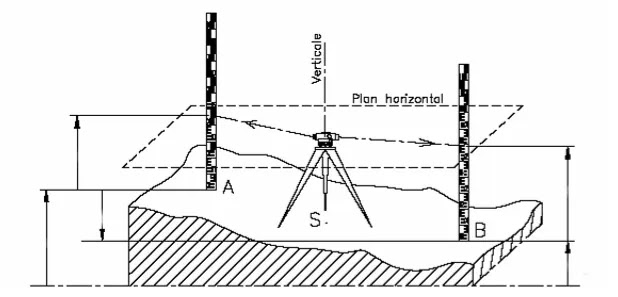Direct leveling consists of determining the height difference ΔHAB between two points A and B using a device : the level and a vertical scale called a staff
The telescopen level consists of a sighting optic rotating around a vertical axis : it therefore defines a horizontal  sighting plane. The target is placed successively on the two point A and B . The operator reads the valus LA on the staff placed at A and the value LB on the staff placed at B.
This drio is an algebraic value whose sign indicates wherher B is higher or lower than A (if ΔHAB is negativee then B is lower than A).
The altitude of a point A notedAlta is the distance counted along the vertical which separates it from the geoind (leveel 0 surface. If the altitude of point A id knoxn,, we can deduce that of point B.

The staff is linear scale which must bee held vertically (it includes a spherical level) on the point involved in the height difference to be measured. The precision of its graduation and its maintenance in a vertical position strongly influence the precision of the height difference measured.A level's reticule is usually made up of four wires:
₪  The leveling wire

The reading on each wire is estimated visually to the nearest millimeter, compared to the example on the previous page:
₪  Upper stadia wire (sup wire) =
₪  Lower stadia wire (lower wire) =
₪  Wire leveler wire level =

The stadia wires make it possible to obtain an approwimate value of the range (horizontal distance between the device and the staff) using the relatioship:
2. The journey

2.1 Simple pathways
When points A and B are located so that a singht (distance, mask,toogreat a difference in height, etx...) the total difference in height must be broken down into elementary differences in level using intermediate points. The set of these decompsitions is called leveling by progression.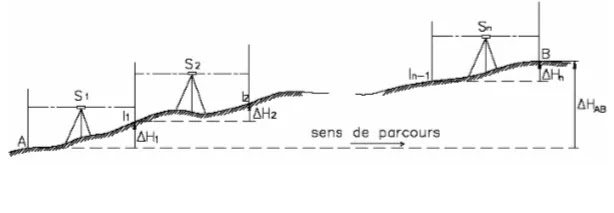A framed path starts from an " origin point' known in altitude, passes through a certain number of inteermediate points and closes on an " end point" different from the point of origin and also known in altitude.
When one seeks to determine the altitude of an end point B from that known of a benchmark A.
when one seeks to determine the altitude of an end point B from that known of a ebnchmark. one generally performs a rounde of B and to check the validity of the measurementsby finding the altitude of A.
When a traverse constitutes a loop returning to its starting point A, it is called a closed traverse.
In all cases, thee calculation principale is as flows:
₪  The staff being on the origin pont 'A', the opertor parks the leveel in ' S1' the distance of which he determines by counting the numbner of steps separating A from S1, so as not to exceed to maximum range of 60m. The operator reads backward, that is to say in the chosen direction of travel, on point 'A', denoted Lar (A)

₪  The staff moves to come to the first intermediate point 'I1' as stable as possible (stone, metal base called 'toad', stake, etc...) and whose distance it determines by counting the number of step separating '  «S1» to « I1 »

₪  Still stationed in 'S1', the operator reads on the staff the front reading on 'I1' denoted Lav (I1); it is then possible to calculate the height difference from 'A' to 'I1' as flows :  :

₪  The operator moves to choose a station 'S2' and so on..

2.2 Applications on simple paths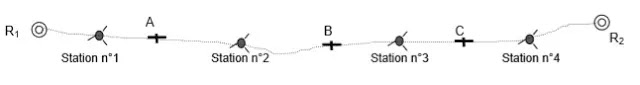Ajouter une légende

 ₪ Depuis la station n°1 on fait les lectures suivantes : ₪ LAR sur R1 = 1,208m    LAV sur A   = 1,312m ₪ Depuis la station n°2 on fait les lectures suivantes : ₪ LAR sur A = 1,735m    LAV sur B = 1,643m ₪ Depuis la station n°3on fait les lectures suivantes : ₪ LAR sur B = 1,810m    LAV sur C = 0,763m ₪ Depuis la station n°4on fait les lectures suivantes : ₪ LAR sur C = 1,739m    LAV sur R2 = 1,934m

Point R1 of known altitude 35,000 NGF
Point R2 of known altitude 35,840 NGF
Determine the altitude of points  A, B et C ?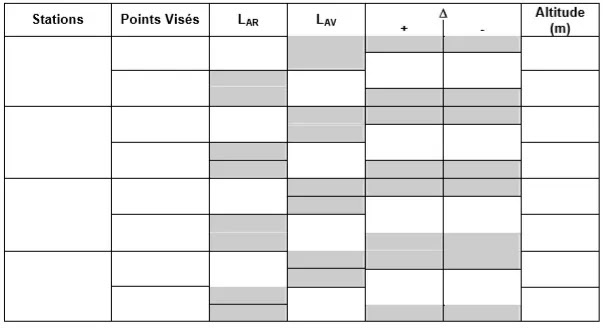2.3 Mixed pathways

From any station of the level in a traverse, and after having recorded the backward reading on the previous traverse point, the operator aims at several detail points and performs on each of them a single reading which is therefore a forward reading.
Then it ends the station by playing forward to the next waypoint. For example, in the figure below, the points 1 , 2 and 3 are radiated from the station S1 whose rear point is the reference (R) and the front point A.
The operation in S1 is called radiation.

When a traverse includes radiated points and traverse points, it is said to be a mixed traverse
The progression of the figure opposite passes through the points R, A, B, C, D, E and R’.  Points 1, 23, 4, 5, 6 and 7 are radiated.
The whole is a  cheminement mixte encadré  between R and R´. on the leveling book, a radiated point is directly identifiable by the fact thatr it does not include a back readingWhen the measurement is finished, the path is first calculated without taking into account the radiated detail points. Then the radiated points are calculated and noted, for exeample; in another color.
Their calculation is fifferent from that of chimney points :
₪  All points radiated from the same station are calculated from the altitude of the back point of the station. This difference in calculation : first the path alone, then the rays and by using different colors.

₪  There is no compensation on the height difference of a radiated topographic point since there is no possible control of its value.

Calculate the altitude of points A, B, Cand D knowing that the leveling mark R is located at a glasses altitude of 38,775 m (NGF.) What is the altitude of the sighting plane ?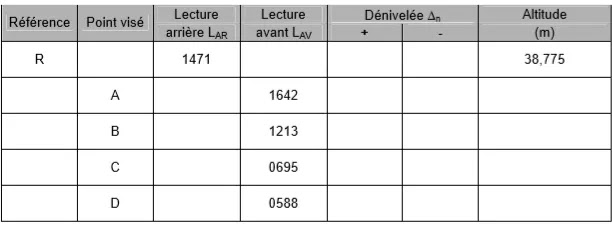2.5 Rules on compensation

As a general rule on a closed and framed path, the altitude of the true reference point is different from the altitude of the calculated point, there is a closing difference that must be compensated for.
The closing gap can come from :
₪  Poor horizontality of the device
₪  A malfunction of the device.

Not knowing the true origin of the closed gap, we established the following rule :

1. The closing deviation is small, i.e. thee deviation is less than the standard deviation, in this case the compensation is proportional to the number of elevation changes2.6 Applications on compensations

Exercise n°1 : We give the diagram of thee following framed progression complete the land leveling book knowing that the altitude of point R1 and AltR1 = 10,354m anand Alt12 = 10,390m.Exercise n°2 : We give the diagram of the following mixed path. Complete the Topography leveling book knowing that the altitude from point R is AltR = 54,870m.

1.